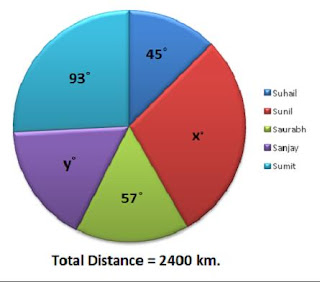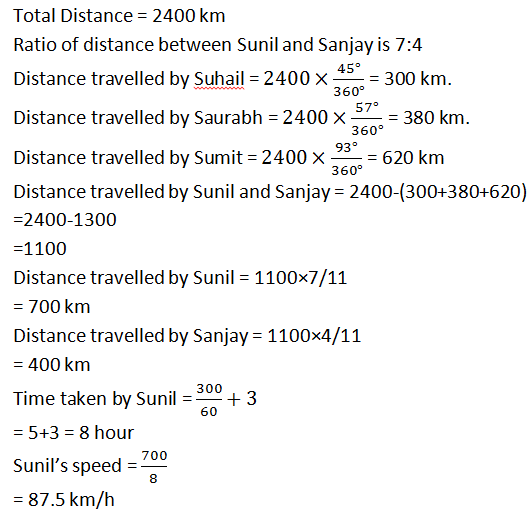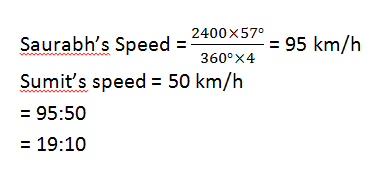# High Level Missing Data Interpretation Set- 15Direction (Q.1-5): Study the following pie-chart and table carefully and answer the questions that follow:

Some values are missing. Answer the questions on the basis of given table and information in question.Person
Speed
Time
Suhail
60 km/h
300 min.
Sunil
-
-
Saurabh
-
4 hour
Sanjay
-
-
Sumit
50 km/h
-

### Question.1

If the time taken by Sunil is 3 hours more than that of Suhail to cover the given distance and the ratio of the distance between Sunil and Sanjay is 7:4, find the Suhail’s Speed.
A. 55 km/h
B. 62 km/h
C. 87.5 km/h
D. 72.5 km/h
E. None of these

Ans. C.
Solution:### Question.2

With the help of question 1 Find the average speed of Sunil and Suhail.
A. 73.75 km/h
B. 62 km/h
C. 50 km/h
D. 76.92 km/h
E. 75 km/h

Ans.D.
Solution:### Question.3

Sumit is going to Delhi with a speed of x km/h. Delhi is 384 km far from his house. If the time is taken by him as same as to travel 324 km with the speed of 15m/s. Find the value of x.
A. 50 km/h
B. 64 km/h
C. 75 km/h
D. 80 km/h
E. 52.5 km/h

Ans. B.
Solution:

According to the question:
Time is taken by Sumit to cover the distance of 384 km with the speed of x = Time taken by Sumit to cover the distance of 324 km with the speed of 15 m/s.### Question.4

If the difference between the speed of Saurabh and Sanjay is 55 km/h and time taken by both is 14 km to cover the given distance. What will be time taken by Sanjay to cover the distance of 250 km.
A. 6.25 hour
B. 5 hour
C. 5.25 hour
D. 7.5 hour
E. None of these

Ans. A.
Solution:= 95km/h.

Time taken by Saurabh is given that is 4 hours.

So time taken by Sanjay is 10 hours.

If the Speed is 95+55 = 150 km/h

Then distance = 150×10 = 1500 km.

But this is not possible because the distance travel by Sunil and Sanjay is 1100 km.

If the distance is 95-55 = 40 km/h

Then distance = 40×10 = 400 km.

So the speed is 40 km/h

Time taken to cover the distance of 250 km =250/40

= 6.25 hour

### Question.5

Find the ratio of the speed between Saurabh and Sumit.
A. 11:8
B. 10:19
C. 15:7
D. 8:11
E. 19:10

Ans. E.
Solution:#### What's trending in BankExamsToday

Smart Prep Kit for Banking Exams by Ramandeep Singh - Download here